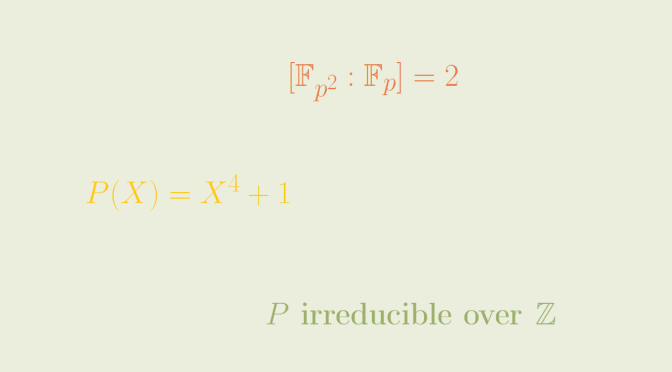An irreducible integral polynomial reducible over all finite prime fields

A classical way to prove that an integral polynomial $$Q \in \mathbb{Z}[X]$$ is irreducible is to prove that $$Q$$ is irreducible over a finite prime field $$\mathbb{F}_p$$ where $$p$$ is a prime.

This raises the question whether an irreducible integral polynomial is irreducible over at least one finite prime field. The answer is negative and:
$P(X)=X^4+1$ is a counterexample.

$$P$$ is irreducible over $$\mathbb{Z}$$

As $$P$$ doesn’t have integer roots, if $$P$$ was reducible it would be the product of two quadratic polynomials:
$P(X)=(X^2+aX+b)(X^2+a^\prime X+b^\prime).$ Hence following equations:
$\left \{ \begin{array}{lcl} b b^\prime & = & 1\\ a^\prime b + a b^\prime & = & 0\\ b + b^\prime +a a^\prime & = & 0\\ a + a^\prime & = & 0\\ \end{array} \right .$ Those equations don’t have solutions over $$\mathbb{Z}$$ as they imply $$b=b^\prime$$, $$\vert b \vert=1$$ and $$a=-a^\prime$$ and therefore $$2=a^2$$ which has no solution over $$\mathbb{Z}$$.

$$P$$ is reducible over all $$\mathbb{F}_p$$ where $$p$$ is a prime

$$P$$ is reducible over $$\mathbb{F}_2$$ as over that field we have:
$P(X)=X^4+1=(X^2+1)^2$ So let’s now suppose that $$p$$ is an odd prime and that $$P$$ is irreducible over $$\mathbb{F}_p$$. The non-zero elements of the finite field $$\mathbb{F}_{p^2}$$ form a multiplicative group $$\mathbb{F}^*_{p^2}$$ that is a cyclic group of order $$p^2-1$$. As $$p$$ is supposed to be odd, it can be written $$p=2r+1$$ and $$p^2-1=4r(r+1)$$. Therefore $$8$$ divides $$p^2-1$$ and $$\mathbb{F}_{p^2}$$ contains an element $$a$$ of order $$8$$.

Considering the polynomial:
$Q(X)=X^8-1=(X^4-1)(X^4+1)$ one can notice that $$a$$ is a root of $$Q$$ and also of $$P$$: $$a$$ cannot be a root of $$X^4-1$$ as it would be of order $$4$$. The multiplicativity formula for degrees gives:
$2=[\mathbb{F}_{p^2} : \mathbb{F}_p ]=[\mathbb{F}_{p^2} : \mathbb{F}_p(a) ][\mathbb{F}_p(a) : \mathbb{F}_p ]$ leading to a contradiction as $$[\mathbb{F}_p(a) : \mathbb{F}_p ] \ge 4$$ if $$P$$ is supposed to be irreducible over $$\mathbb{F}_p$$.